# Stress (mechanics) facts for kids

Kids Encyclopedia Facts
(Redirected from Tensile stress)

Mechanics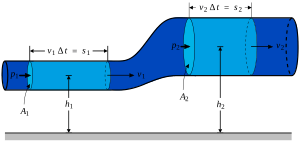Solid mechanics
Solids
Stress · Deformation
Compatibility
Finite strain · Infinitesimal strain
Elasticity (linear) · Plasticity
Bending · Hooke's law
Failure theory
Fracture mechanics
Frictionless/Frictional Contact mechanics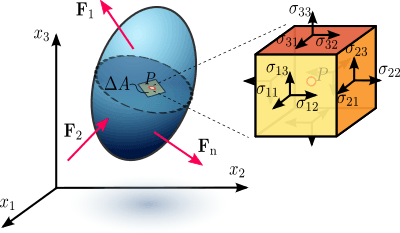Figure 1.1 Stress in a loaded deformable material body assumed as a continuum.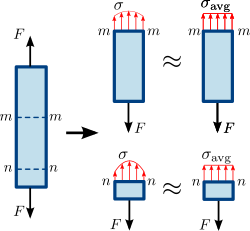Figure 1.3 Normal stress in a prismatic (straight member of uniform cross-sectional area) bar. The stress or force distribution in the cross section of the bar is not necessarily uniform. However, an average normal stress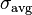$\sigma_\mathrm{avg}\,\!$ can be used.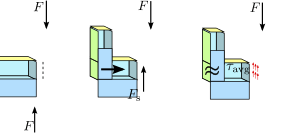Figure 1.4 Shear stress in a prismatic bar. The stress or force distribution in the cross section of the bar is not necessarily uniform. Nevertheless, an average shear stress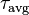$\tau_\mathrm{avg}\,\!$ is a reasonable approximation.

Stress is the force per unit area on a body that tends to cause it to change shape.

Stress is a measure of the internal forces in a body between its particles. These internal forces are a reaction to the external forces applied on the body that cause it to separate, compress or slide. External forces are either surface forces or body forces. Stress is the average force per unit area that a particle of a body exerts on an adjacent particle, across an imaginary surface that separates them.

The formula for uniaxial normal stress is: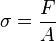${\sigma}=\frac{F}{A}$

where σ is the stress, F is the force and A is the surface area.

In SI units, force is measured in newtons and area in square metres. This means stress is newtons per square meter, or N/m2. However, stress has its own SI unit, called the pascal. 1 pascal (symbol Pa) is equal to 1 N/m2. In Imperial units, stress is measured in pound-force per square inch, which is often shortened to "psi". The dimension of stress is the same as that of pressure.

In continuum mechanics, the loaded deformable body behaves as a continuum. So, these internal forces are distributed continually within the volume of the material body. (This means that the stress distribution in the body is expressed as a piecewise continuous function of space and time.) The forces cause deformation of the body's shape. The deformation can lead to a permanent shape change or structural failure if the material is not strong enough.

Some models of continuum mechanics treat force as something that can change. Other models look at the deformation of matter and solid bodies, because the characteristics of matter and solids are three dimensional. Each approach can give different results. Classical models of continuum mechanics assume an average force and do not properly include "geometrical factors". (The geometry of the body can be important to how stress is shared out and how energy builds up during the application of the external force.)

## Simple stresses

In some situations, the stress within an object can be described by a single number, or by a single vector (a number and a direction). Three such simple stress situations are the uniaxial normal stress, the simple shear stress, and the isotropic normal stress.

### Uniaxial normal stress

Tensile stress (or tension) is the stress state leading to expansion; that is, the length of a material tends to increase in the tensile direction. The volume of the material stays constant. When equal and opposite forces are applied on a body, then the stress due to this force is called tensile stress.

Therefore in a uniaxial material the length increases in the tensile stress direction and the other two directions will decrease in size. In the uniaxial manner of tension, tensile stress is induced by pulling forces. Tensile stress is the opposite of compressive stress.

Structural members in direct tension are ropes, soil anchors and nails, bolts, etc. Beams subjected to bending moments may include tensile stress as well as compressive stress and/or shear stress.

Tensile stress may be increased until the reach of tensile strength, namely the limit state of stress.

## Stress in one-dimensional bodies

All real objects occupy three-dimensional space. However, if two dimensions are very large or very small compared to the others, the object may be modelled as one-dimensional. This simplifies the mathematical modelling of the object. One-dimensional objects include a piece of wire loaded at the ends and viewed from the side, and a metal sheet loaded on the face and viewed up close and through the cross section.

## Images for kidsStress (mechanics) Facts for Kids. Kiddle Encyclopedia.